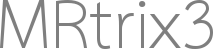# Vector plot tool - Plot primary orientation described by smallest eigenvalue

Dear experts,

I am working on a model in which the primary orientation is described by the eigenvector corresponding to the smallest eigenvalue (basically the opposite case of CSD).
I find mrview a great tool to visualize rapidly gradient orientations and I would like to use the “vector plot tool” for this purpose with my model.
Can anyone please tell me how to make this modifications on the source code (or where to start) to adapt this tool (vector plot tool) to my purpose?

Best,
Liz

You don’t need to make any modifications to the source code. The vector plot tool will display any 4D image, interpreting the first 3 volumes as the [ X Y Z ] components of the vector. So assuming you were using the `dwi2tensor | tensor2metric` combination, you could get the smallest eigenvector with a command like:

``````dwi2tensor dwi.mif - | tensor2metric - -num 3 -vector vec.mif
``````

i.e. using the `-num 3` option to select the smallest eigenvalue/vector.

If you’re producing images from your own model, you’d just need to provide this type of 4D image (whether in `.mif` or NIfTI format), and load it in the tool.

1 Like

Thank you very much for your help and clear explanation. Exactly what I was looking for.

Liz